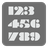Is Even
Elementary
English PL RU

Check if the given number is even or not. Your function should return True if the number is even, and False if the number is odd.

Input: An int.

Output: A bool.

Example:

```is_even(2) == True
is_even(5) == False
is_even(0) == True
```

How it’s used: (math is used everywhere)

Precondition: both given ints should be between -1000 and 1000